# Numerical Reasoning Question Formulae

We have created the this page to assist you in your preparation for your numerical reasoning test. In order to succeed in your upcoming psychometric tests there are some basic formulae you may need.

#### Percentage change question

The percentage change between two numbers describes how much the original figure needs to change by to become the new figure.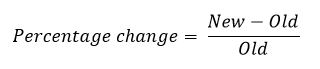In order to calculate this in your numerical reasoning test, you will need to take the old number away from the new number and then divide the result by the old number.

For example if the number of shoes sold in february and march was 38 and 51 respectively the percentage change would be calculated as follows: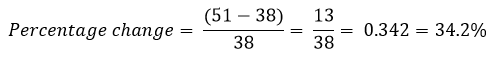#### Ratio questions

A ratio describes the relationship between two figures. For example the number of parts required to mix a particular colour of paint. If 8 litres of blue paint and 12 litres of yellow paint are required to make 20 litres of a particular shade of green paint, the ratio of blue to yellow is 8 to 12.

This can be simplified as 2 parts blue to 3 parts yellow. Ratios can be displayed in different ways, this also could have been shown as 2:3 or 2/3.

#### Order of operations - BODMAS

During your numerical reasoning test you will probably need to use many basic operators such as multiplication, division, addition and subtraction. The order in which you perform these operations can sometimes affect the result. In school you may have been taught to remember this as BODMAS!

1. Brackets - Always perform the operation inside the brackets first (using the BODMAS principle inside)
2. Order - Next is to perform and powers or square roots
3. Division and Multiplication - Division and multiplication is next (in any order)
4. Addition - Working left to right
5. Subtraction - Working left to right

We have an example below to show the range of possible answers that you could get if you perform the operations in the incorrect order. Try this for yourself!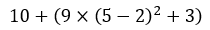As there is more than one operation, BODMAS tells us we need to start with the brackets.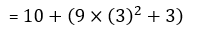We refer to BODMAS again and go inside the second set of brackets.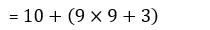Next we perform the orders, i.e. 3 squared.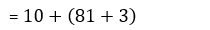Staying inside the brackets, we perform the multiplication.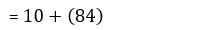We can then add the numbers inside the brackets.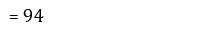Collapsing the brackets and performing the final addition, gives the answer.

If for example we decided to ignore BODMAS after step 2 (i.e. after the second line) the answer could have been very different.Lets start at step 3 again.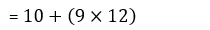Ignoring BODMAS, we could decide to do the addition next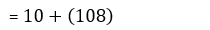Now we could start following BODMAS again and complete the operations in the brackets.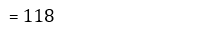Collapsing the brackets and adding gives you a very different answer to the above.

As you can see, it is important to follow the BODMAS principle when there are multiple operations. The order of the operations matters in mathematics and so will have a big impact on your numerical reasoning test.

#### Practice makes perfect

In order to prepare for your numerical reasoning tests it is important that you practice. This will ensure that you are prepared for the types of question you may face and how you may go about answering them.